# Assignment Result - Groups

My second assignment on groups was marked and returned. As predicted, there's a lot to take away from this.

## Basic Groups

At this level it seems it's not sufficient to use notation like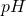,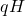and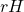to refer to subgroups. In my head, I know what the binary operator of these subgroups is but for the benefit of the reader the (better) convention is to be explicit with the binary operator: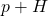,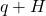, and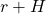specifically.

Definition check: a cyclic group is just a group that contains a generator that generates the whole group. It doesn't have to "cycle" back to the beginning of the ordered set. eg, infinite sets can form a cyclic group under addition.

Mistakes in basic proofs. This one is a classic. With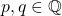, I had to prove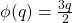was onto. My answer: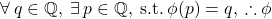Which I was all smug about because it looks pretty. But this is just the definition of the function being onto and I'd neglected to state the actualthat always exists. Namely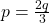.

## Group Classification

Group decompositions can't be written as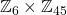. It needs to be written as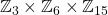. 3 and 15 are not coprime, so cannot be combined.

## Finite Groups

As a part of a group presentation, there are kind of identities like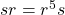. However, for the most part in your answer, especially when you're talking directly about elements being in your group, they must always be in the form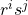(with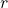first).

In being asked to deduce an isomorphism, I heavy-handedly defined both groups (as they were small) and then also stated a function explicitly that would define the isomorphism. Apparently it's just enough to state that both groups were of order 2. Therefore they're isomorphic.

I need to become a lot more familiar with the concept of centres of a group. To show that a subgroup is the centre of its parent group, I needed to show that the subgroups elements were centres of the subgroup. I was was just trying to wing it, and although I essentially got the answer right, I showed I had a complete misunderstanding of centres.

# Chapter 10 of 24 - Quadratic Reciprocity

Fun chapter. Squares are very important, turns out. Chapter was about methods of solving quadratic formulas modulo some prime.

Covered Euler's Criterion for quadratic residues, The Legendre symbol, Gauss's Lemma, and then the law of quadratic reciprocity.

I'll be looking at rings next. I've been waiting to investigate these things for a while...

# Chapter 9 of 24 - Multiplicative Functions

Multiplication! That sounds nice and simple doesn't it! Unfortunately, that's not what that title means.

Any number can be broken down into its prime decomposition (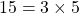). A multiplicative function is where a function of a number is equal to the function of each prime in the prime decomposition of that number all multiplied together. So if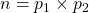, where p is a prime in the prime decomposition and if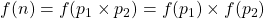, then the function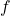is a multiplicative function.

Next section went into detail about perfect numbers. A perfect number is equal to the sum of its positive factors excluding itself. Best example here is the perfect number 6=1+2+3.

The rest of the chapter was introducing Euler's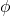-function (also multiplicative), and using this new function, the introduction of primitive roots.

# Chapter 8 of 24 - The Sylow Theorems

Yet another confusing chapter that I couldn't spend enough time in. Overall, the Sylow Theorems just relate to subgroups of prime, and prime-power, order. Very generally, you can use the Sylow Theorems themselves to determine isomorphism and whether or not a group is a direct product of other cyclic groups.

That was the last of group theory! I'm about to submit my group theory assignment, and I can tell I'll have a lot to write about once that is returned. Next up, is the third book of the set, "Numbers and Rings".